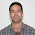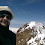## Thursday, September 13, 2018

### P = NP and Cancer

Often when the question comes to what happens if P = NP, one typically hears the response that it kills public-key cryptography. And it does. But that gives the impression that given the choice we would rather not have P = NP. Quite the opposite, P = NP would greatly benefit humanity from solving AI (by finding the smallest circuit consistent with the data) and curing cancer. I've said this before but never explained why.

Of course I don't have a mathematical proof that P = NP cures cancer. Nor would an efficient algorithm for SAT immediately give a cancer cure. But it could work as follows:
1. We need an appropriately shaped protein that would inhibit the cancer cells for a specific individual without harming the healthy cells. P = NP would help find these shapes perhaps just the DNA of the person and the type of cancer.
2. At this point we don't understand the process that takes a ACGT protein sequence and describes that shape that it forms. But it must be a simple process because it happens quickly. So we can use P = NP to find a small circuit that describes this process.
3. Use P = NP to find the protein sequence that the circuit from #2 will output the shape from #1.
We'll need an truly efficient algorithm for NP problems for this to work. A n50 algorithm for SAT won't do the job. All this steps may happen whether or not P = NP but we'll need some new smart algorithmic ideas.

Please note this is just a thought exercise since I strongly believe that P ≠ NP. I do not want to give false hope to those with friends and loved ones with the disease. If you want to cure cancer your first step should not be "Prove P = NP".

1.Ill-informed articles such as this one are the reason why Trump got elected.

2.Why is it ill-informed? Elaborate ...

3.n-body simulation is PSPACE-complete and thus no guarantee that P=NP (even a linear time algorithm) would mean we can simulate the folding of proteins quickly (https://en.wikipedia.org/wiki/N-body_simulation#Computational_complexity).

And even if we could, no guarantee that we can actually so precisely target cancer cells using just proteins in the first place.

4.So we keep mentioning the efficiency of the algorithm in terms of the input size (n), but what defines (n) for SAT ? Is it the number of clauses ? Is is the number of total variables ? Which one of them ?

1.As with most decision problems, complexity for SAT is conventionally measured in terms of the length of the input. This is roughly O(clauses * log(variables)). Some authors use 'n' for the number of variables though, so in actual papers you want to check the definitions. It rarely matters in casual contexts, since SAT problems with very low or very high clause/variable ratios are generally easy.

2.Ty, that really helped.

5.This article sounds just about as ridiculous to biologists as it does to quantum computing folks when people with no background talk about their field...

6.You could see my proof of UP=NP in

and see my proof of P = NP in

For UP=NP, we have

https://zenodo.org/record/1419750#.W55I5xnB8ew

For P=NP, we have

https://zenodo.org/record/1420486#.W5_TC9JKjIU

In general, you could see my whole research in

7.How many variables are in those problems?
(We do have kind of efficient SAT solvers.
But don't we need NP=LIN to handle those bio problems?)

8.So according to Ryan Williams on Twitter SAT ist solvable now, but the next level of the PH requires exponential time. I guess this is not proven, just a new ETH. History might repeat itself, and maybe in 15 years Sigma2 SAT is solvable in practice and cancer will be cured after all.

9.The point being made is valid. A similar statement was proved a few years ago: that "physics is hard". That means if P=NP physics would be easy, with all of the engineering benefits of that.

There is, however, a question about P=?NP that I have never seen seriously addressed, and I think should be. Bluntly, does the human brain solve NP problems, and does it do it in P?

There are obvious technical issues with measuring how much computational work the brain does, but are there any non-trivally-small-N NP problems that the human brain is known to solve? Are problems like face recognition and such known to be in, or not in, NP? Does the brain, at best, only do a random "paratrooper" solution to non-convex problems, and arguably with an exponential amount of work?

It would be interesting to know if A: not even the human brain can solve NP problems in P, or, alternatively, that the human brain does solve NP problems in P. One would think that that is an actual testable empirical fact that could be found out.

Again, there are all sorts of technical problems to answering that question. One could say that Sudoku problems are NP, but that the human brain arguably supplies an exponential amount of power on them. One could also say that a lot of apparent NP problems (like Sudoku) are solved by human brains by relying on an exponential amount of memory of solved problems, and that the actual computation is minor. There is also the issue that the human brain my have trillions of cells, but that only a small percentage are devoted to actual computation, so judging the amount of power available is tricky. Still, the question should be answerable, and the answer would be interesting.

There is also the problem of measuring the computational size in analogue systems. A (hypothetical) true Real space system has an arguably infinite computational capability. That is what makes solving problem with physical simulations so hard to use as computational models. To the extent that the brain uses continuously variable signals that would be another problem in looking at what problems the brain can solve and how much work it does in solving them.

A look at animal models might be interesting, as they present smaller systems. Can an earthworm solve a (very small) NP problem? If no, is there a threshold in brain size that can?

The obvious joke is that if the human brain could solve NP problems in P, it would have already settled the P=?NP issue. But that does not answer the question of if the human brain does solve some NP problems. A yes or no to that question would make the P=?NP question more interesting.

10.See my complete poof of UP = NP on

https://zenodo.org/record/1433419#.W6eHVRnB8ew

11.You could see my proof of UP=NP in

and see my proof of P = NP in

For UP=NP, we have

https://zenodo.org/record/1434273

For P=NP, we have

https://zenodo.org/record/1434277

In general, you could see my whole research in

12.You could see my proof of UP=NP in

and see my proof of P = NP in

1.13.14.